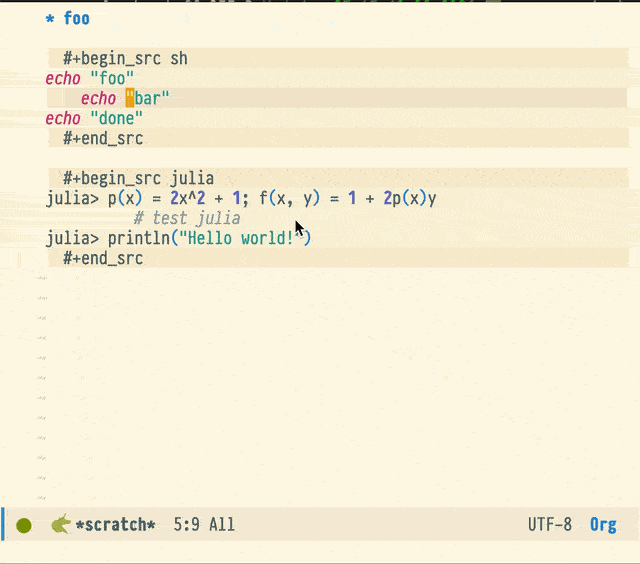# [org] 如何缩进一堆代码块内的代码，有没有常用的发布样式？

``````#+HTML_HEAD: <link rel="stylesheet" type="text/css" href="http://www.pirilampo.org/styles/readtheorg/css/htmlize.css"/>

``````

``````(setq org-edit-src-content-indentation 0)
``````

`C-x (` 开始定义，`C-x )` 结束定义，`C-x e` 执行宏，之后连续 `e` 可以连续执行

`C-x (`

`C-c '`

`C-x h`

`<TAB>`

`C-c '`

`C-c C-v C-n`

`C-x )`

`C-x e e e e e e e `

@ringawho 好麻烦啊，其实我想参考子龙山人的代码，虽然这个配置在`org-mode`下好像不起作用

``````(defun indent-region-or-buffer()
(interactive)
(save-excursion
(if (region-active-p)
(progn
(indent-region (region-beginning) (region-end))
(message "Indent selected region."))
(progn
(indent-buffer)
(message "Indent buffer.")))))

(global-set-key (kbd "C-M-\\") 'indent-region-or-buffer)
``````

org-indent-block，不过需要挪到 begin_src/end_src 那一行操作。可以写个命令，带上 org-previous-block ，应该能满足缩进的需求

``````(defun claude/org-indent-block (arg &optional block-regexp)
"Indent the block around point."
(interactive "p")
(org-previous-block arg block-regexp)
(org-indent-block))
``````

`C-x (` 开始定义键盘宏

`C-c '` 编辑源码块

`C-x h` 选中所有文本 （这个刚刚忘说了，抱歉，我再更改一下前面的回复）

`<TAB>` 格式化

`C-c '` 退出编辑源码块

`C-c C-v C-n` 跳到下一个源码块

`C-x )` 结束键盘宏的定义

1 个赞

``````(defun claude-org/org-indent-block (arg &optional block-regexp)
"Indent the block around point."
(interactive "p")
(let ((current-point (point)))
(org-previous-block arg block-regexp)
(org-indent-block)
(goto-char current-point)))

(if (org-in-block-p '("src"))
(claude-org/org-indent-block arg)
(funcall func arg)))

``````

`org-indent-block`这个函数好像不起作用啊，所以我对这个方案还是有点犹豫的，没去尝试``````(defun claude-org/org-indent-block (arg &optional block-regexp)
"Indent the block around point."
(interactive "p")
(let ((current-point (point)))
(org-previous-block arg block-regexp)
(org-indent-block)
(goto-char current-point)))

(if (org-in-block-p '("src"))
(claude-org/org-indent-block arg)
(funcall func arg)))

``````

org-indent-block 基于 org-indent-region，你可以试一下手动选择代码块，执行 org-indent-region ，如果这也不能正常缩进，那就属于另外的问题了

org升级到9.4之后代码块内的编辑方式变得不可捉摸。。

pandoc是命令行工具。使用下面的代码实现文档转换：

``````pandoc 源文档.md -o 目的文档.org
``````

pandoc的安装你bing一下就能找到。很方便的。官方文档写的也非常清楚。如果需要可以在官网查看。